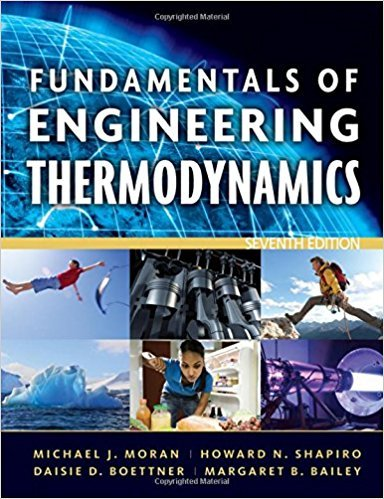×
×

# Using Energy Concepts and the Ideal Gas ModelTwo-tenthsISBN: 9780470495902 50

## Solution for problem 138P Chapter 3

Fundamentals of Engineering Thermodynamics | 7th Edition

• Textbook Solutions
• 2901 Step-by-step solutions solved by professors and subject experts
• Get 24/7 help from StudySoup virtual teaching assistantsFundamentals of Engineering Thermodynamics | 7th Edition

4 5 0 395 Reviews
23
2
Problem 138P

Using Energy Concepts and the Ideal Gas Model

Two-tenths kmol of nitrogen (N2) in a piston-cylinder assembly undergoes two processes in series as follows:

Process 1−2: Constant pressure at 5 bar from V1 = 1.33 m3 to V2 = 1 m3.

Process 2−3: Constant volume to p3 = 4 bar.

Assuming ideal gas behavior and neglecting kinetic and potential energy effects, determine the work and heat transfer for each process, in kJ.

Step-by-Step Solution:

Step 1 of 3</p>

We are required to calculate the work and heat transfer.

Step 2 of 3</p>

The p-V diagram for the given process can be shown as below.Process 1-2,

This process occurs at constant pressure.

The work done at constant pressure and changing volume process is,Given data:barN/m2m3m3

Therefore,JkJ

Given,kmol

From,KK

From the relation,KK

For the process 2-3,KK

Therefore, from table A-23 for nitrogen,

At temperature 300 K,kJ/kmol

At temperature 400 K,kJ/kmol

The heat transferred in process 1-2 is,kJkJ

Therefore, the heat transferred in the process 1-2 is  -582 kJ.

Step 3 of 3

##### ISBN: 9780470495902

Unlock Textbook Solution